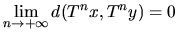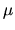## Asymptotic pairs in positive-entropy systems

Ergod. Th. & Dynam. Syst., 22, 671-686, 2002.

### Abstract

We show that in a topological dynamical system (X,T) of positive entropy there exist proper (positively) asymptotic pairs, that is, pairs (x,y) such that x is not equal to y and. More precisely we consider a T-ergodic measureof positive entropy and prove that the set of points that belong to a proper asymptotic pair is of measure 1. When T is invertible, the stable classes (i.e., the equivalence classes for the asymptotic equivalence) are not stable under T -1: for-almost every x there are uncountably many y that are asymptotic with x and such that (x,y) is a Li-Yorke pair with respect to T -1. We also show that asymptotic pairs are dense in the set of topological entropy pairs.

Paper: [arXiv:1901.00327] [pdf]